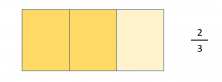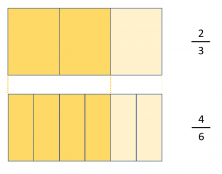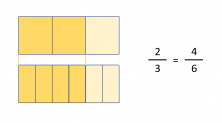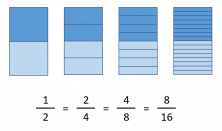Smartick is an advanced online program that teaches kids math and coding in only 15 min. a day

Jul29

# Do You Know What an Equivalent Fraction Is?

#### Do you know what an equivalent fraction is?

We have written about equivalent fractions many times on the blog:

Today, we are going to start by investigating the etymology, or meaning, of the word ”equivalent.” Let’s split the word in two: equi – valent. If you think for a moment, you’ll notice that ”equi” means ”something that is equal” and the second part, ”valent,” means ”value.” By putting the two parts together we get the meaning of equivalent – ”of equal value.”

Therefore, we can conclude that equivalent fractions are fractions that have the same value and… when do two fractions have the same value?

Let’s use a fraction as a reference, 2/3 of a lemon tart.Now, let’s try to think of what other fraction we could have and still eat an equivalent amount of pie.Of course… if we split each portion of the previous fraction in two, we obtain twice the amount of portions as before. In turn, we get a new fraction but we eat the same amount.We went from 2/3 to 4/6, which are two fractions that represent the same amount with a different number of parts.

In this way, we can say that equivalent fractions are those fractions that represent the same amount, even if the numerator and denominator are different.

I guess you won’t be surprised to know that more than two fractions can be equivalent to one another, look!As you can see in the image, 1/2 = 2/4 = 4/8 = 8/16. They are all fractions that represent the same amount and therefore, they are equivalent fractions among themselves.

Now you know what an equivalent fraction is and where its name comes from. If you would like to practice this and other types of fractions, log on to Smartick and sign up for our free trial.